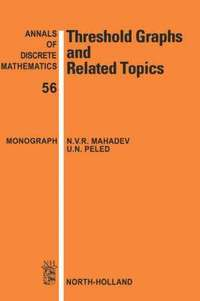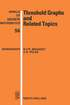Fler böcker inom
Format
Inbunden (Hardback)
Språk
Engelska
Antal sidor
542
Utgivningsdatum
1995-09-01
Förlag
North Holland
Medarbetare
Peled, U.N.
Illustrationer
graphs, bibliography, indexes
Volymtitel
Volume 56
Dimensioner
234 x 156 x 32 mm
Vikt
962 g
Antal komponenter
1
Komponenter
52:B&W 6.14 x 9.21in or 234 x 156mm (Royal 8vo) Case Laminate on White w/Gloss Lam
ISSN
01675060
ISBN
9780444892874# Threshold Graphs and Related Topics

Inbunden,  Engelska, 1995-09-01
1492
• Skickas från oss inom 7-10 vardagar.
• Fri frakt över 249 kr för privatkunder i Sverige.
Kan levereras innan julafton
Finns även som
• Läs i DRM-kompatibel e-boksläsare.
718
Threshold graphs have a beautiful structure and possess many important mathematical properties. They have applications in many areas including computer science and psychology. Over the last 20 years the interest in threshold graphs has increased significantly, and the subject continues to attract much attention. The book contains many open problems and research ideas which will appeal to graduate students and researchers interested in graph theory. But above all Threshold Graphs and Related Topics provides a valuable source of information for all those working in this field.

## Passar bra ihop

1.2. +
3.De som köpt den här boken har ofta också köpt Breath av James Nestor (häftad).

## Kundrecensioner

Har du läst boken? Sätt ditt betyg »

## Innehållsförteckning

Preface. Basic Terminology. Threshold Graphs. Motivation. Basic characterizations. Minimizing integral weights. Perfect graphs and algorithms. Threshold and split completions. Longest cycles and Hamiltonicity. Total coverings and total matchings. Ferrers Digraphs and Difference Graphs. Introduction. Ferrers digraphs, characterizations. The Ferrers dimension. Difference graphs. Degree Sequences. Graphical degree sequences. Threshold sequences. The polytope of degree sequences. Difference sequences. Applications.Introduction. Aggregation of inequalities. Synchronization. Cyclic scheduling. Guttman scales. Split Graphs. Introduction. Basic properties. Hamiltonian split graphs. The splittance of a graph. The Threshold Dimension. Introduction. Bounds for the threshold dimension. Dimensional properties. Operations preserving the threshold dimension. Restricted threshold dimension. NP-Completeness. Introduction. The partial order dimension. Related NP-complete problems. Other complexity results. The split dimension. Polar graphs. 2-Threshold Graphs. Introduction. Properties of 2-threshold graphs. Bithreshold graphs. Strict 2-threshold graphs. Recognizing threshold dimension 2. Recognizing difference dimension 2. Intersection threshold dimension 2. The Dilworth Number. Introduction. Graphs of Dilworth number 2. The Dilworth number and perfect graphs. Box-Threshold Graphs. Introduction. Elementary properties. A transportation model. Frames of BT graphs. Matroidal and Matrogenic Graphs. Introduction. Matroidal graphs. Matrogenic graphs. Matrogenic sequences. Domishold Graphs. Introduction. Notation and main results. Equidominating graphs. Pseudodomishold graphs. The Decomposition Method. Introduction. The canonical decomposition. Domishold graphs and decomposition. Box-threshold graphs and decomposition. Matroidal and matrogenic graphs and decomposition. Pseudothreshold and Equistable Graphs. Introduction. Pseudothreshold graphs. Equistable graphs. Threshold Weights and Measures.Introduction. Threshold weights. Threshold measures. Threshold and majorization gaps. Threshold Graphs and Order Relations. Introduction. Biorders. Bidimensions. Relations of bidimension 2. Multiple semiorders. Enumeration. Introduction. Enumeration of threshold graphs. Enumeration of difference graphs. Extremal Problems. Introduction. Large interval and threshold subgraphs of dense graphs. Maximizing the sum of squares of degrees. Other Extensions. Introduction. Geometric embeddings of graphs. Tolerance intersection graphs. Universal threshold graphs. Bibliography. List of Notations. Author Index. Index.